Latest Banking jobs   »   Reasoning Ability Quiz For IBPS Clerk...

# Reasoning Ability Quiz For IBPS Clerk Prelims 2021- 12th August

Directions (1-2): Study the information carefully and answer the questions given below.
Point U is 3m west of point G, which is 8m north of point V. Point V is 4m east of point B. Point B is 9m south of point H. Point H is 2m west of point L. Point L is 6m north of point C. Point C is 7m east of point M.

Q1. What is the shortest distance between point U and Point M?
(a) 2√18m
(b) √61m
(c) √63m
(d) 8m
(e) None of these

Q2. In which direction point C with respect to point V?
(a) North
(b) North-west
(c) North-east
(d) South-west
(e) None of these

Directions (3-5): Study the information carefully and answer the questions given below.
Point G is 5m west of point H, which is 12m south of point D. Point A is 20m east of point B. Point F is 10m west of point E. Point C is 15m east of point D. Point F is 8m north of point G. Point E is 16m south of point A.

Q3. What is the direction of point G with respect to point A?
(a) South-east
(b) North-west
(c) South-west
(d) West
(e) None of these

Q4. What is the shortest distance between point H and point E?
(a) 8m
(b) √89m
(c) 10
(d) √15m
(e) None of these

Q5. What is the direction of point B with respect to point C?
(a) East
(b) North
(c) North-west
(d) South-East
(e) None of these

Directions (6-8): Study the information carefully and answer the questions given below.
In a family of eight members there are three married couples and five female members, G is daughter-in-law of B, who has two children. D is the aunt of C, D is unmarried. F is father-in-law of H and is married to E. A is the father of D.

Q6. How is H related to E?
(a) Son
(b) Grand father
(c) Son in law
(d) Daughter
(e) Husband

Q7. Who among the following is father of D?
(a) A
(b) F
(c) E
(d) G
(e) B

Q8. How is D related to G?
(a) Daughter-in-law
(b) Sister-in-law
(c) Sister
(d) Nephew
(e) Daughter

Q9. Pointing to a woman, Ravi said, “She is the daughter of my grandfather’s only child”. How is woman related to Ravi?
(a)Daughter
(b) Aunt
(c) Mother
(d) Sister
(e) None of these

Q10. Amit is the son of Shiv. Shiv’s sister, Jaya has a son Rohit and a daughter Yami. Riya is the mother of Amit’s father. How is Yami related to Riya?
(a) Mother
(b) Granddaughter
(c) Sister
(d) Niece
(e) None of these

Q11. E is the son of A. D is the son of B. E is married to C. C is B’s daughter. How is D related to E?
(a) Brother
(b) Uncle
(c) Father-in-law
(d) Brother-in-law
(e) None of these

Directions (12-14): Study the information carefully and answer the questions given below.
Seven persons i.e. P, Q, R, S, T, U and V are in the family of four-generation with one married couple. There are three female members of the family. V is the mother of T, who is grandmother of S. R is son of the one who is daughter in law of P. R has two children. S is female and she is not sister of Q.

Q12. Who among the following is grandson of V?
(a) U
(b) T
(c) R
(d) Q
(e) None of these

Q13. Who among the following is mother-in-law of Q?
(a) P
(b) V
(c) R
(d) S
(e) U

Q14. How is U related to T?
(a) Grandson
(b) Granddaughter
(c) Father
(d) Mother
(e) None of these

Q15. If ‘P + Q’ means ‘P is the father of Q’, ‘P × Q’ means P is the brother of Q’; ‘P – Q’ means ‘P is the mother of Q’, then which of the following is definitely true about X – Z + Y?
(a) Y is the son of Z
(b) Z is the son of X
(c) Y is the father of Z
(d) X is the mother of Y
(e) None of these

Solutions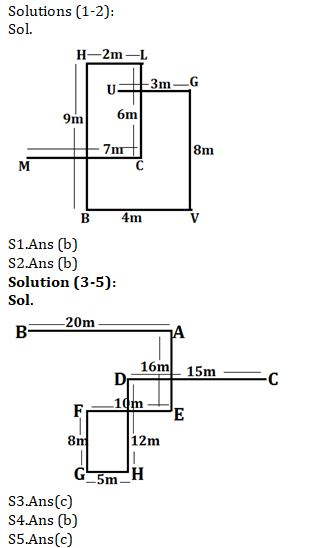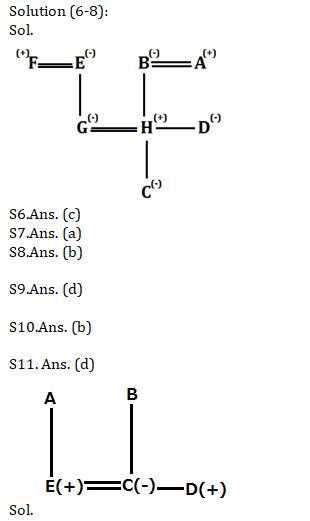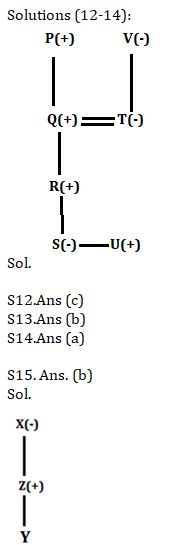#### Congratulations!Incorrect details? Fill the form again here

•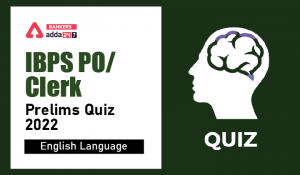English Quizzes For IBPS Clerk/PO Prelim...
•English Quizzes For IBPS Clerk/PO Prelim...
•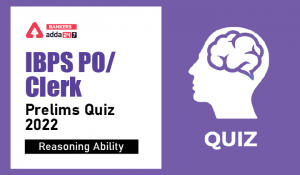Reasoning Ability Quiz For IBPS Clerk/PO...
•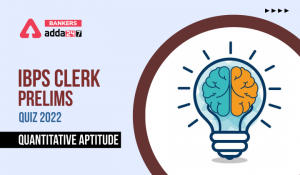Quantitative Aptitude Quiz For IBPS Cler...
•Quantitative Aptitude Quiz For IBPS Cler...
•Reasoning Ability Quiz For IBPS Clerk/PO...